# 动态规划

2.阅读代码——动态规划

## 斐波那契

### 1.递归算法

void fun(int n)
{
if(n==0)
return 0;
if(n==1)
return 1;
reurn fun(n-2)+fun(n-1);
}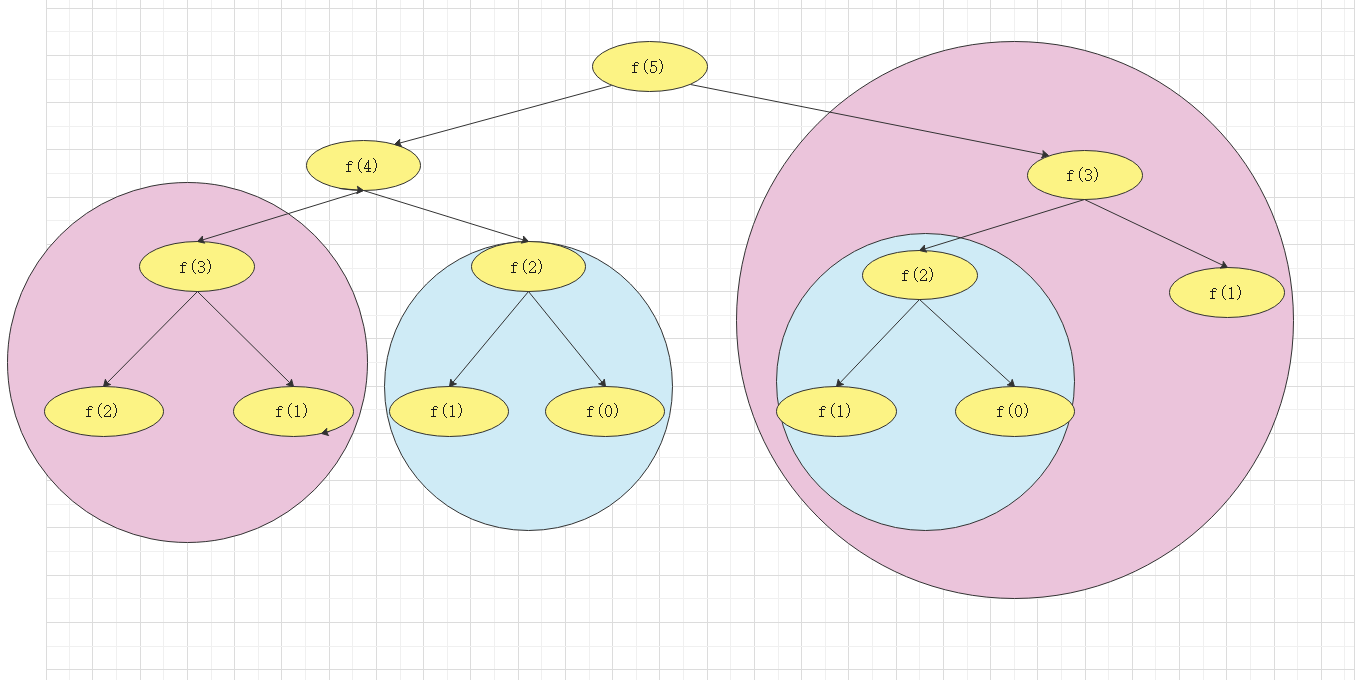## 3.动态规划的典型应用

### 一维应用：

int fun(int x)
{
int dp[max];
dp=0;dp=1;dp=1;

for(int i=3;i<=x;i++)
{
dp[i]=dp[i-1]+dp[i-2];
}
return dp[x];
}


## 题目1：给你一个字符串，如19216801这一个字符串，通过在任意位置加入三个点，组成合理的ip地址，请问，它有几种合理的方案

• 合理的ip地址 X.X.X.X,,并且每一位都必须小于255，拆成四位。
• ip拆成四位，数字不能以0开头。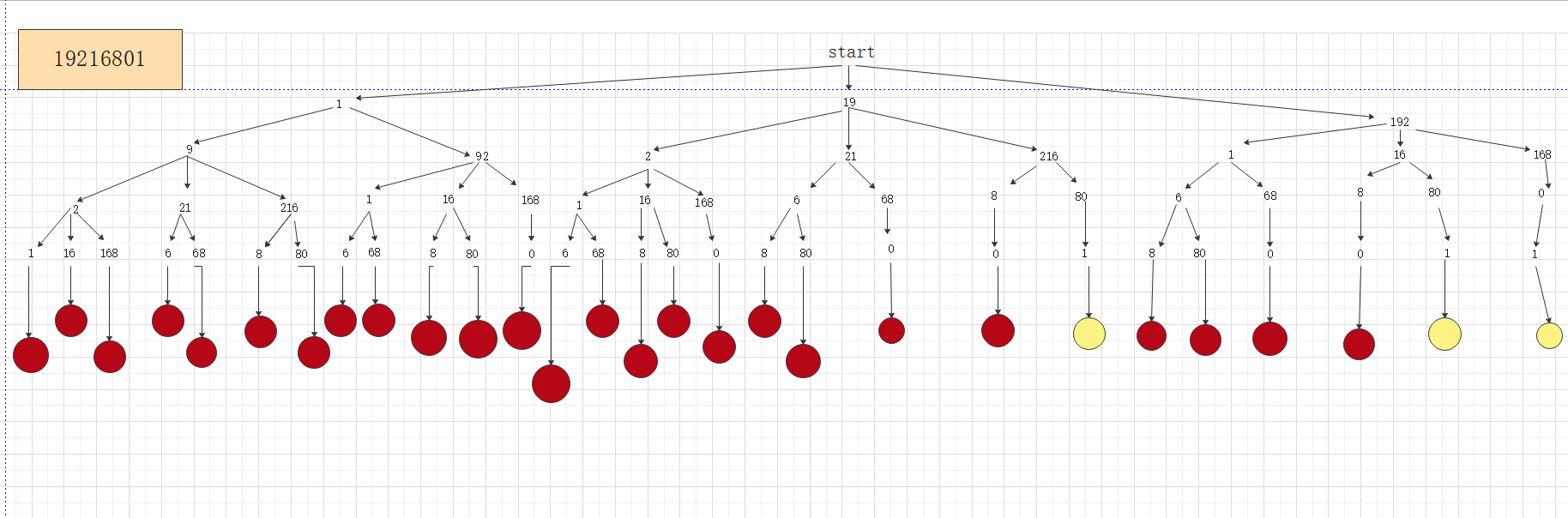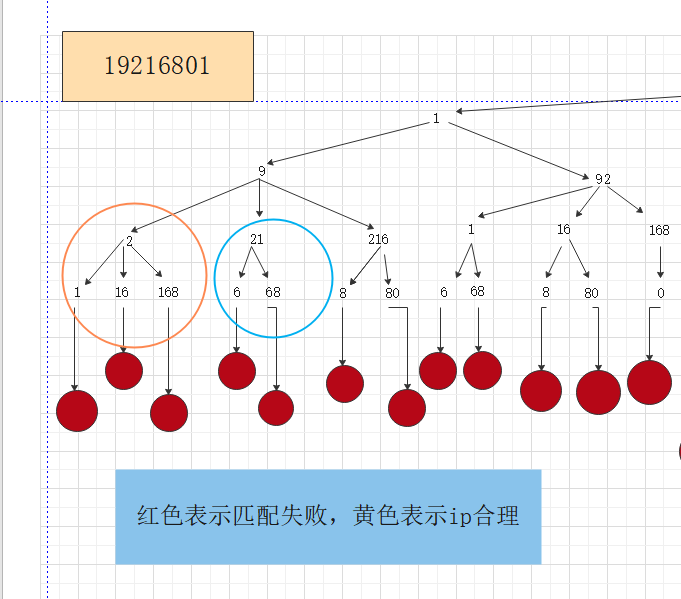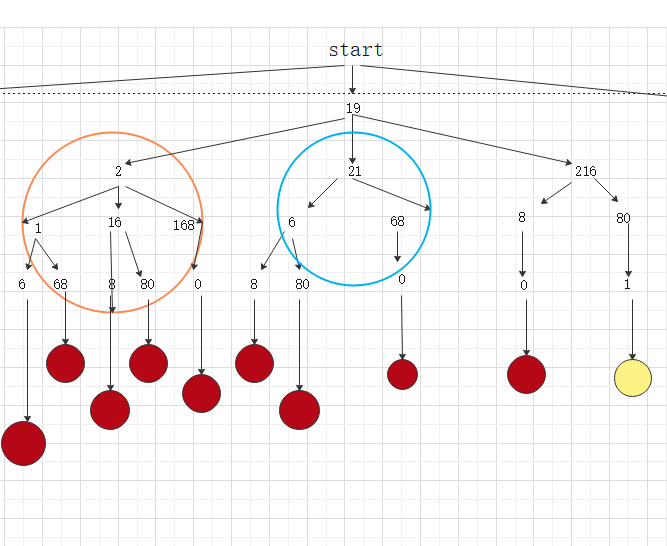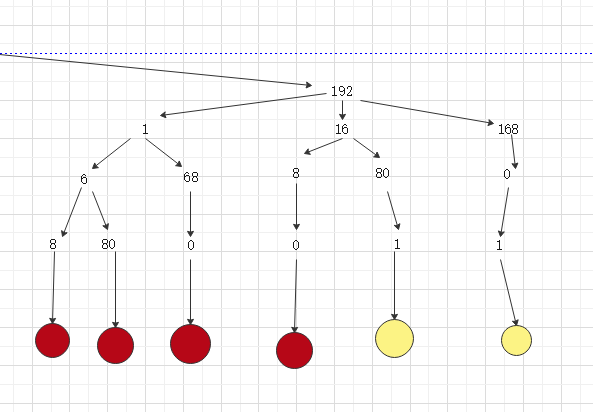• 由图一和图二的橙色圈圈和蓝色圈圈可知，则这道题画成一棵树，则会出现很多的重复子问题，那么用动态规划的图如下，这道题，要选择二维dp数组作为载体，来存放已解决的子问题。

• 画图注意点：

• 1.对于一个动态规划的问题，一定要理解dp[]或dp[] []对应的含义，例如在斐波那契数列里，dp[x]就表示斐波那契数列的值，而在这道题里，我们把题目进行分解，要求将字符串拆成四个255以内的值，那么dp[] [j]就可以前j个字符串，dp[i] []则表示可以表示i个255以内的值，合起来的意思，就是前j个字符串可以表示i个255以内的值
• 2.在画图的时候，其实也要注意，为了让我们对题目更好的理解，我们一般会多申请一个空间，从1开始。
• 3.我们题目所要的解一般在d[max] [max]这个地方，这个题目例题要我们求的就是d ,str前8个字符能组成几种255以内的数

动态规划图解分解过程：

第一步：对d[i] [j]数组进行初始化，对i和j等于0的地方，可赋值为0，因为没有意义；

比如i=0时，j=1时，表示1可以表示0个255的方法有几种，显然没意义，所以直接赋值为0；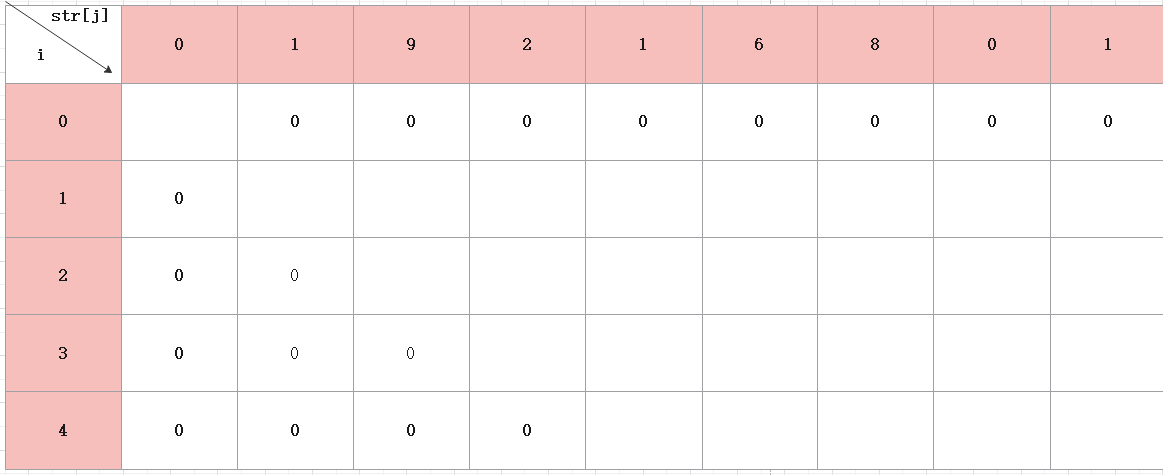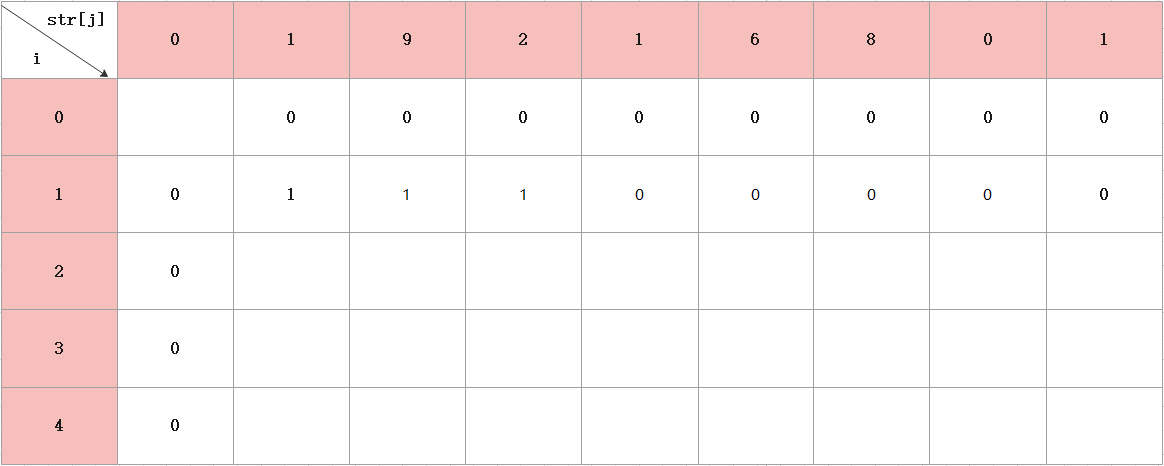• 从j=1开始看，将字符串1表示成1个255以内的数，有一种
• j=2，将字符串19表示成1个255以内的数，有一种
• j=3，将字符串192表示成1个255以内的数，有一种
• j>=4开始，就会看到已经是四位数了，大于255，所以是0

• 由前面可知，j=1时，根据意义，1不能表示2个255以内的数，所以是0

• j=1时，要注意，从i=2开始后，不是直接看19是255以内的数，那它就是一种，这种想法是错误的，对于递归问题转动态规划，我们要看它的起源，所以j=2，先看9是不是255以内的数，则应该返回i=1那一行看，如果9是，则d +=d ,然后再看19是不是255以内的数，如果是，则d +=d ，所以最终d =1.

•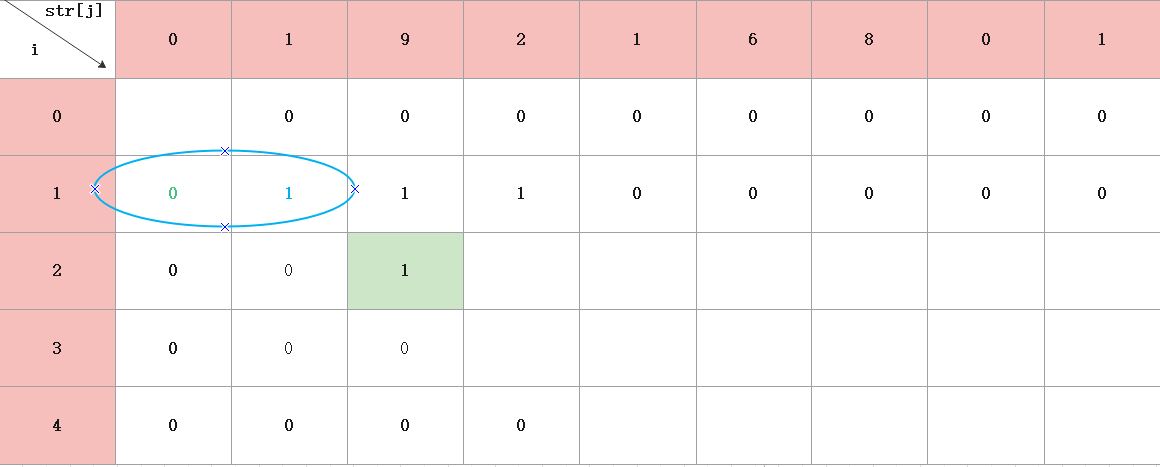•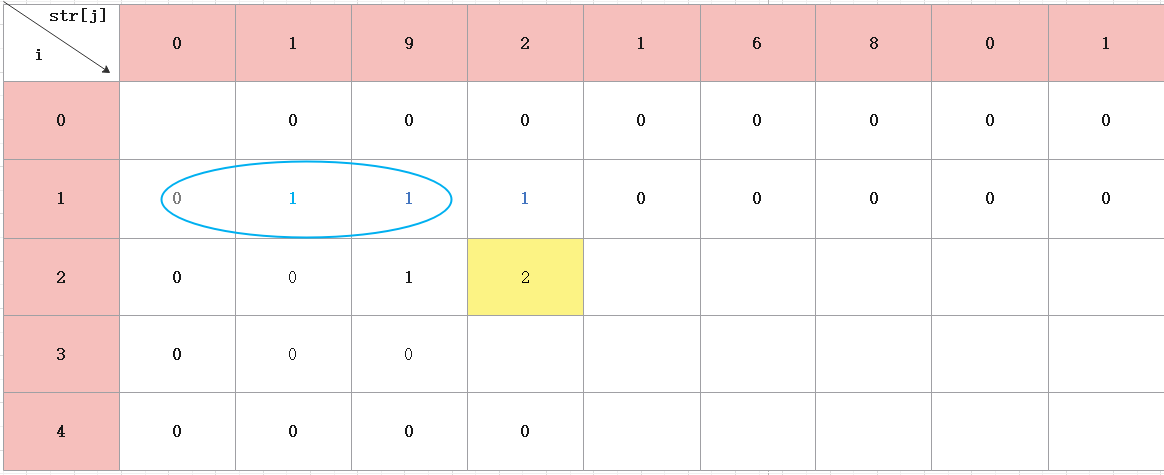d +=dp ，看192是不是255以内的数，如果是d +=dp 。最终，d =2。

• 同理，后面的数，也是这样得出。经过多次运算，我们会发现一种规律，每一个格子的值都是当前格子左上角的三个格子之和组成，那为什么是三个格子，也不一定，看具体要求，因为这道题是不超过255，那肯定只能是三位数，所以就是三个格子。最后，组成的图如下：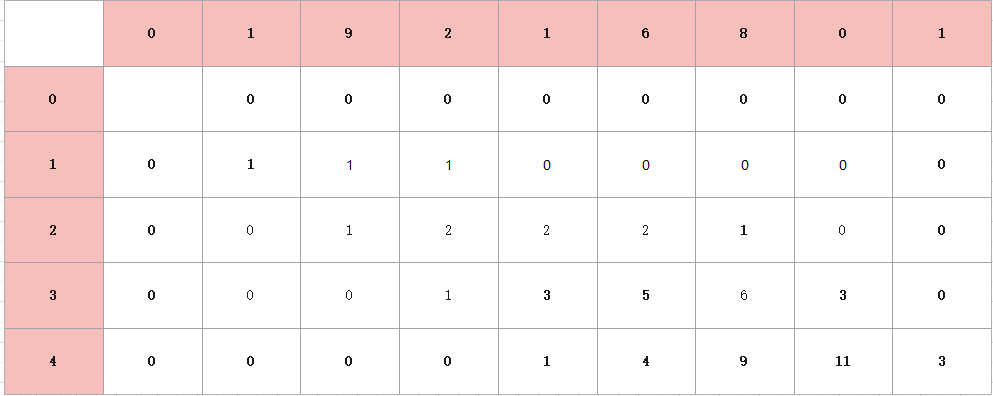• 那么我们会有一个疑问，为什么最后的答案就是我们要求。这需要我们回归树形图。拿其中一个格子来说。

•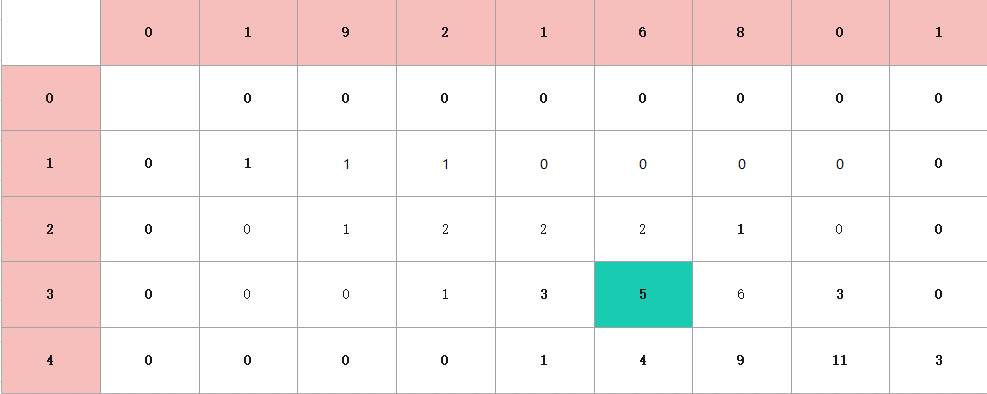• 为什么这个格子是5？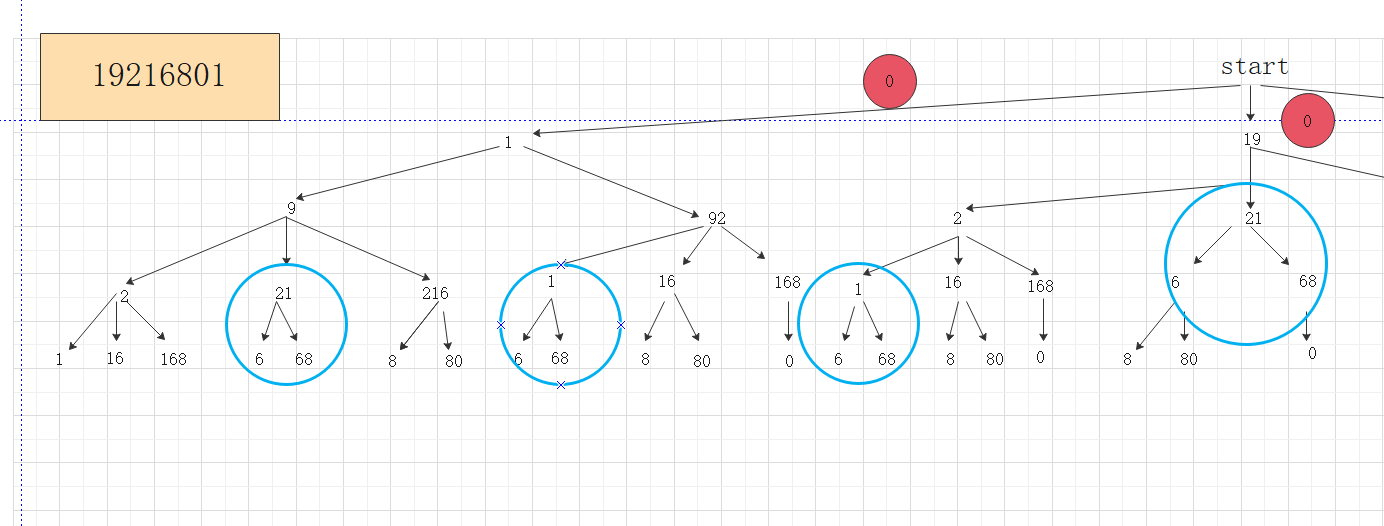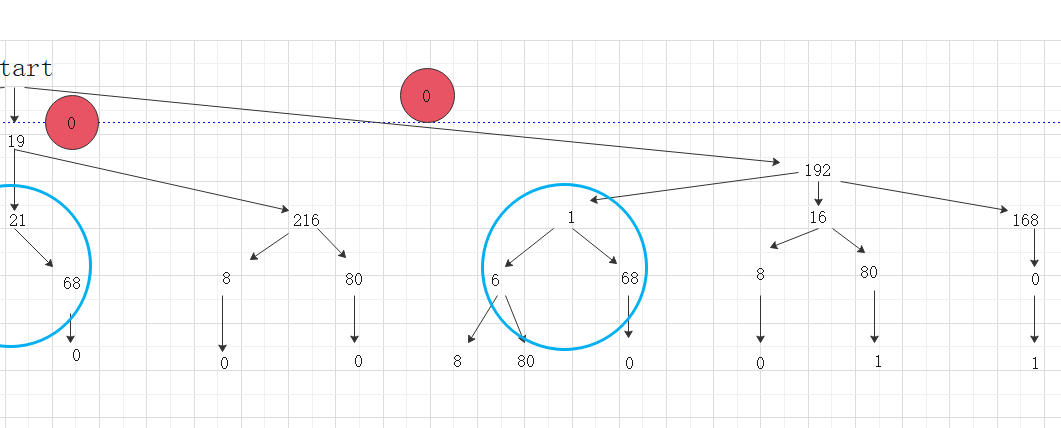• 仔细数数，图中蓝色的圈圈是否有5个，就说明6的起源有5个，再回归方格图，我们就会有一种感觉，如果看的是dp =3这个数，我们就可以去树形图里找1的起源，会有三个圈，而1所在的树的层次肯定会比6的层次高，因为1在前面，所以1会是6的子问题，所以，这棵树，与这个表结合，表从上到下的子问题的答案，到最后，我们要求的答案，而树，从上到下，每一个分支，也是一个子问题，到叶子节点，可能就是我们要找的答案。

#### 动态规划代码：

int DP(string str)
{
int len=str.length;
int dp[len+1]={0};
//进行初始化
for(int i=0;i<5;i++)//先对dp数组进行遍历赋值
{
for(int j=i;j<len;j++)
{
if(i==0&&j==0)//dp置为1，后面比较好算
{
dp[i][j]=1;
continue;
}
if(i==0)//第一行没有意义，所以为0
{
dp[i][j]=0;
continue;
}
dp[i][j]=0;
for(int x=1;x<=3;x++)//三位数
{
if(j-x>=0&&validate(str.substr(j-x+1,x)))
//str.substr(a,b),表示从j-x+1这个地方开始截取x个字符
//validate()这个函数是判断数字
{
dp[i][j]+=dp[i-1][j-x];
}
}

}
}
return dp[len]
};
int validate(string str)
{
if(s=='0')  return true;
if(s.startWith('0')) return false;
return parseInt(s)<=255;
}
/*

str.startWith(ch)    //判断字符串是否以‘0’开头
parseInt(str)      //是将str字符串转化为数字的函数
*/


#### 伪代码

int DP(string str)
{
得到字符串的长度len
申请多一个空间的dp数组，并初始化

for i=0 to i=4//对dp数组进行遍历
{
for j=i to j=len-1
{
if(i==0&&j==0)//dp置为1，后面比较好算，不影响之前的分析
{
dp[i][j]=1;
continue;
}
if(如果是第一行)
{
没有意义，则dp[i][j]=0;
continue;
}
dp[i][j]=0;
for x=1 to x=3  //三位数
{
if(从j-x+1这个地方开始截取x字符，并且数字有意义)
{
dp[i][j]+=dp[i-1][j-x];
}
}

}
}
}


#### 运行结果：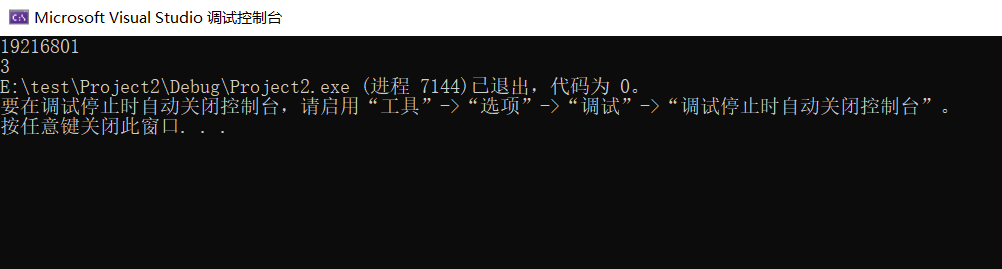## 题目二：• 这道题，一开始看着题目有点像是哈夫曼树求最小权值，后来发现是不一样的，这道题要求是相邻的，哈夫曼树没有这个条件，所以代码放进去是错误的。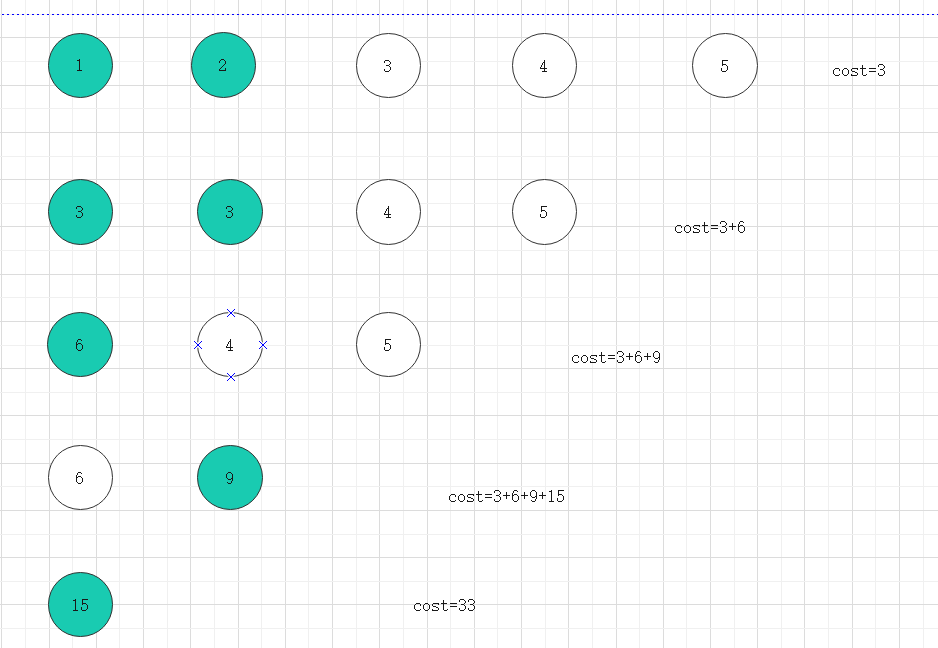#### 解题思路：

• 步骤一，计算sum[]数组，表示第i堆石子到第j堆石子的总和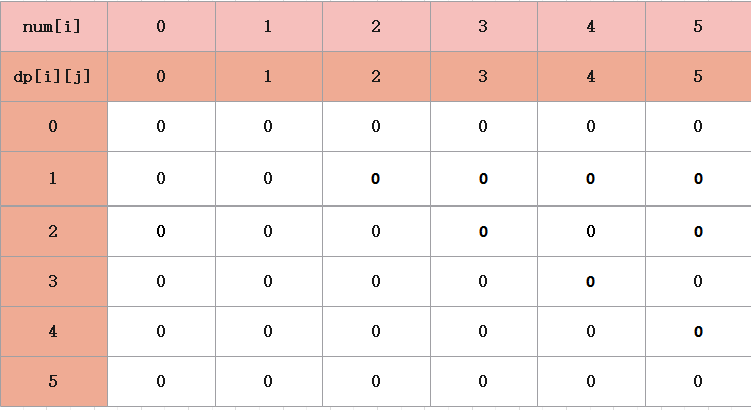• 对sum[i]数组里，我们可以横着看每一行，i=1时，从j=1开始，代表的意义就是如果从第一个石子往右开始合并，先不计较到底要怎么合并花费比较小，从第二行开始，代表的意义就是从第二个石子开始从左往右合并，不考虑开销，这样，就可以罗列出所有合并的可能性，那么我们得出所有可能性后，要做的就是，让这些可能性进行关联，找出最小开销。
• 那么对下面第二行，第三行也是同理的。

#### 代码实现：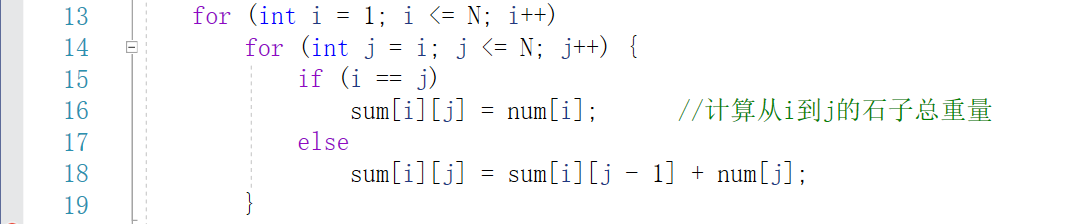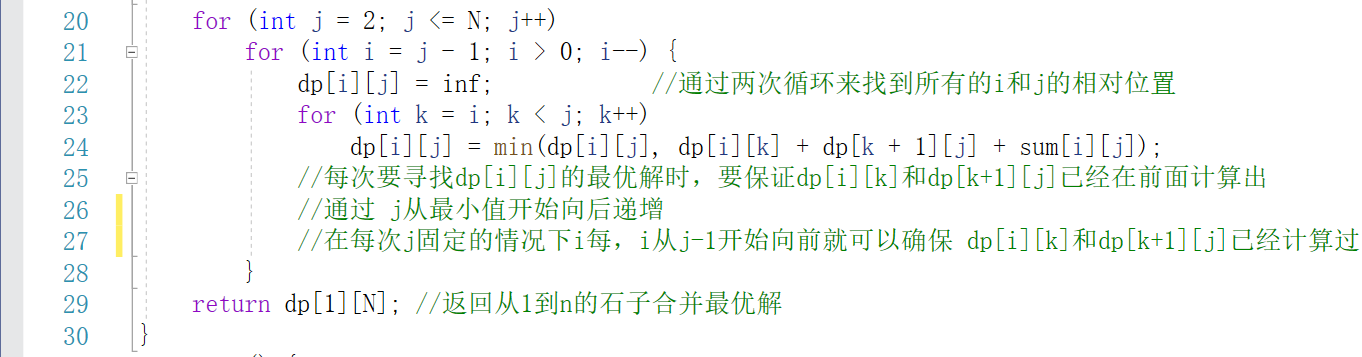#### 伪代码：

for i=1 to i=N
for j=i to j=N
计算把第i堆合并到第j堆需要的费用
用sum数组存储
for j=2 to j=N
{
for i=j to j=0
{
先对dp[i][j]初始化为无穷大
for k=i to k=j-1
{
从i到j共有j-i个状态,取最优值
dp[i][j]=min(dp[i][j],dp[i] [k]+dp[k+1][j]+sum[i][j]);
}
}
}


#### 运行结果：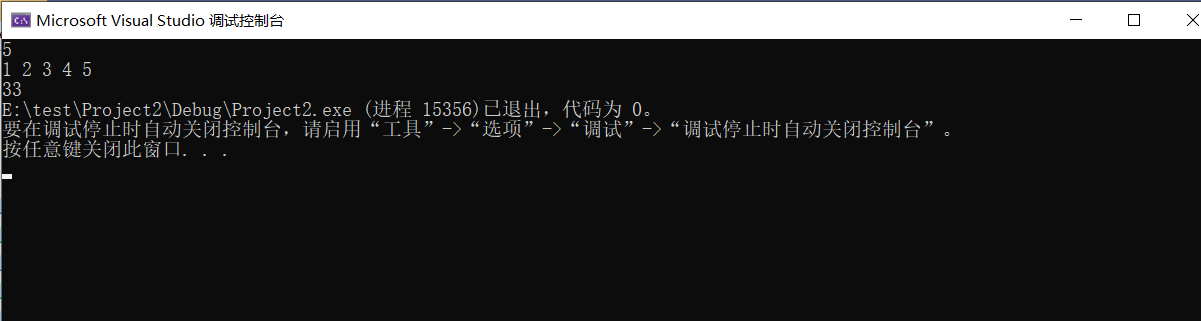DP过程：

j=2,i=1

j=3,i=2

j=3,i=1

j=4,i=3

j=4,i=2

j=4,i=1

#### 图解如下：

• 首先，对dp[i] [j]进行初始化。

• 对于动态规划，我们要知道，最核心的部分，就是能够找到dp[i] [j]横坐标，纵坐标表示的含义。

• 对于此题，dp[i] [j]表示的含义其中一种是就是从第i堆合并到第j堆的最小代价。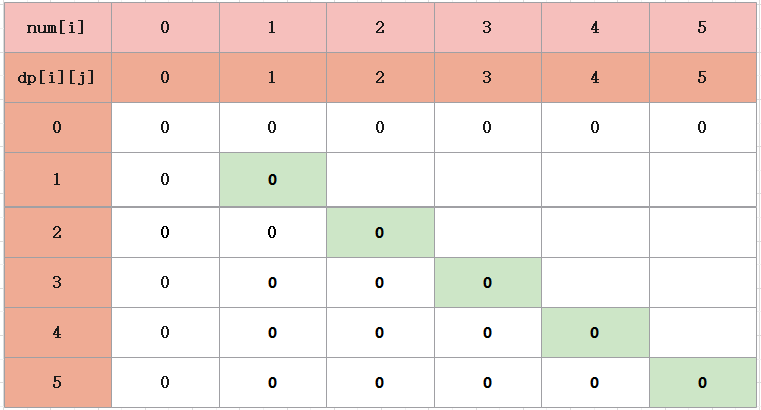• j=i，表示自己合并自己，不用花费任何钱
• 第二步，将两堆合并成一堆需要的费用。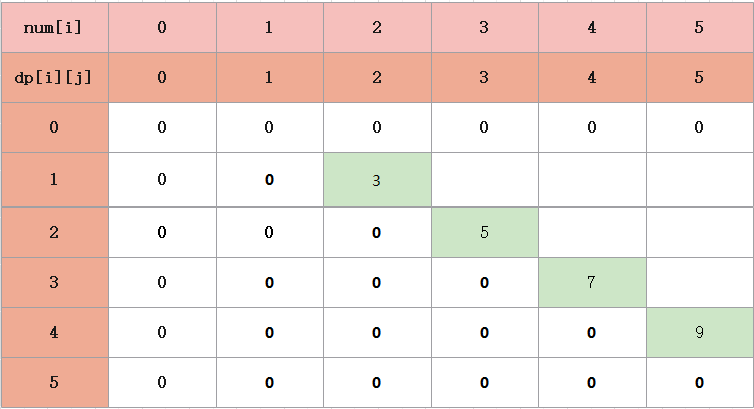• 第三步，将三堆合并成一堆，这里可能会感到疑惑的是为什么是三堆，我们已经解决了相邻两堆之间的问题，那么在三堆之间，我们则要对当前对进行分析，是要选右边的并成一堆，还是选左边的并成一堆，所以，肯定是选和比较小的，所以还记得我们之前做的准备sum数组嘛，它记录了每一堆从左往右要花费的钱钱，这时，它就起了作用。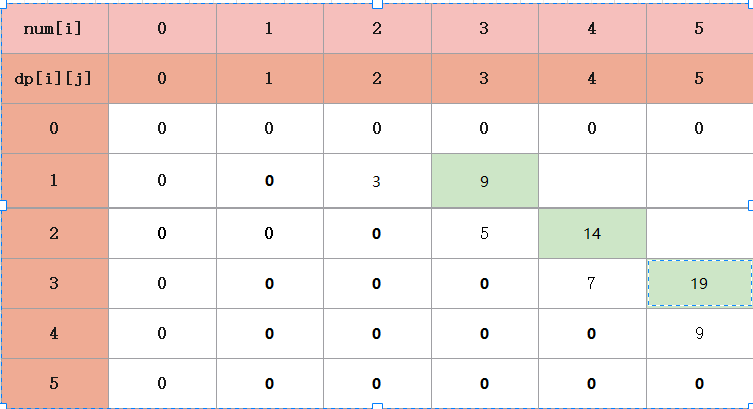• 对于i=1，j=3这个点来说,它的含义就是将第一堆（1），第二堆（2），第三堆（3）合并在一起，那么总的费用就是第一堆和第二队进行合并cost1=3，12堆和3进行合并，就是cost2=3+3=6，所以总的费用就是3+6=9。
• 那么下面对于i=2，j=4也是同理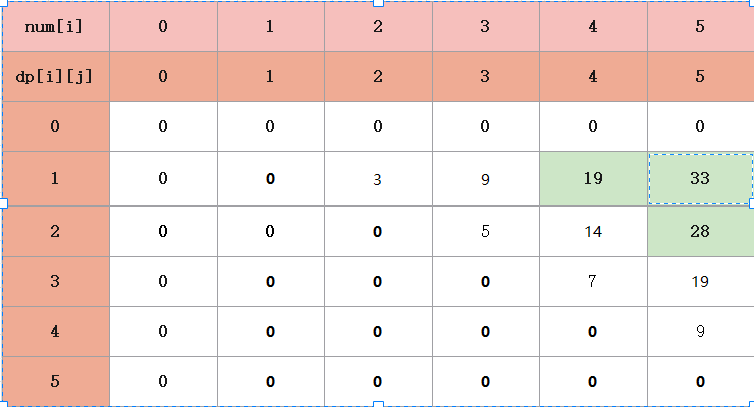• 我们来举个例子吧

• 看表里的i=2，j=4,结合代码，含义是把第二堆第三堆第四堆进行合并

• k=2时，dp +dp +sum 

• k=3时，dp +dp +sum 

• 从上面就可看到，dp 有两种选择，第三堆和第四堆先合并，再和第二堆进行合并，或者第二堆和第三堆先合并，再合并第四堆，然后比较它们之间的大小，就可以求出正解

# 题目三：环形相邻两堆石子合并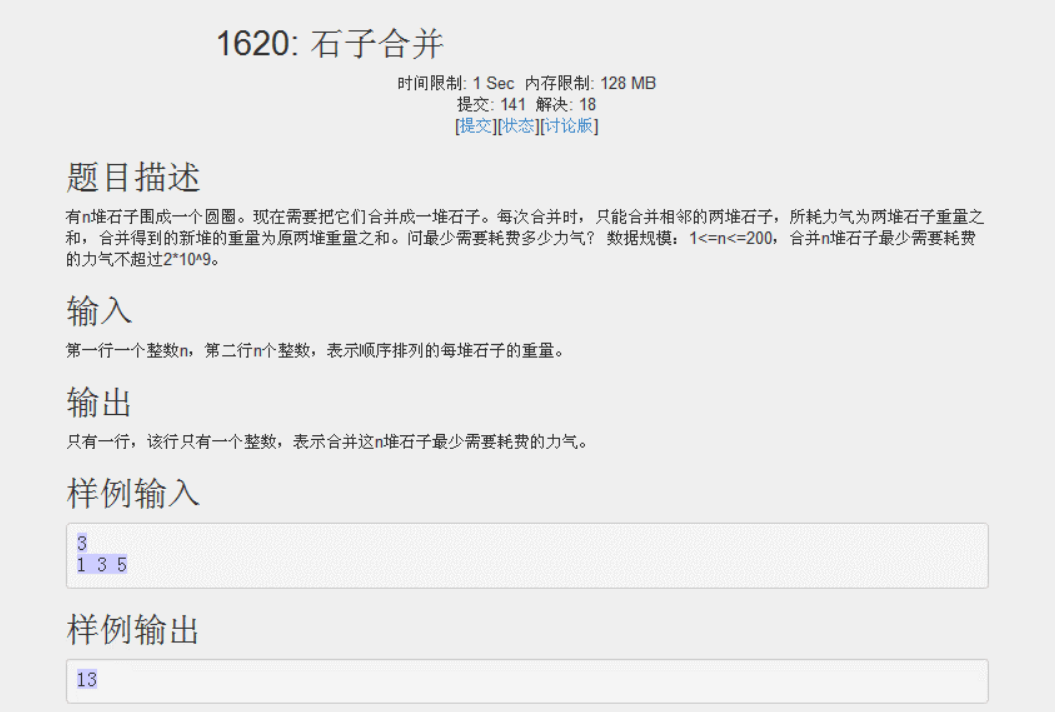#### 解题思路：

• 环形结构，经常采用双倍长度线性化的手段，也就是说，把环形结构看成是长度为环的两倍的两倍的线性结构来处理，将环化成线性结构

• 环的长度是N，所以题目相当于与有一排石子，1，，，，N,N+1，，，2N,然后就可以用线性的石子合并问题的方法做了

• 有个地方需要注意，f（i,j)总是和f(N+i,N+j)相等，所以可以减少一些不必要的计算。

• 将N结构的线性表，转换成双倍的2N结构的长度。然后在2N长度的表中，截取我们需要的长度N的部分就可以了

• dp[i] [j]表示从第i堆合并到第j堆（合并成一堆）的最小代价

• sum[i]表示前i堆石子的和

• 状态转移方程：

• dp[i] [j]=min(dp[i] [k]+dp[(i+k+1)%n] [j-k-1]+sum[i] [j])(0<k<=j-1)(j<=k<j)

dp过程：

• len...1>n len表示归并长度
• i...1>2n i表示起点
• 那么j=i+len-1
• k...i>j-1

#### 代码实现：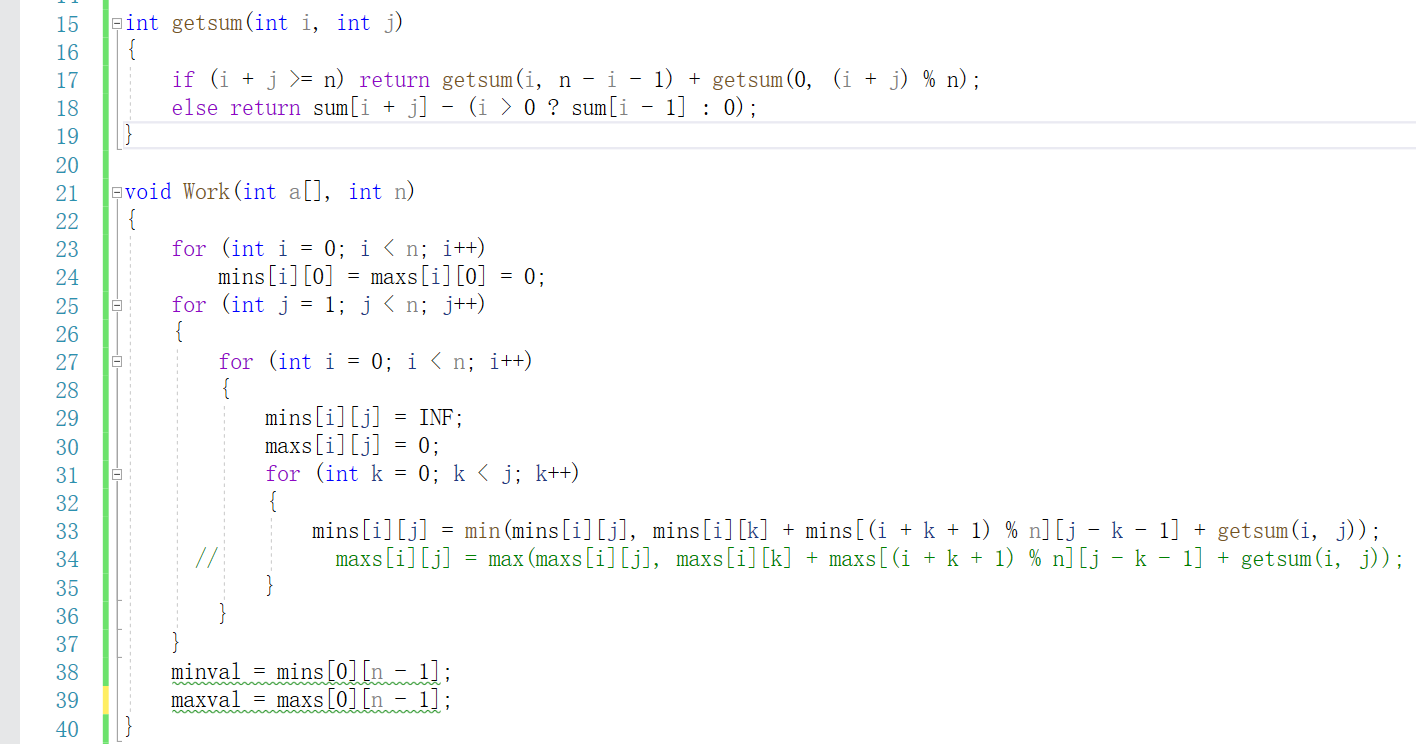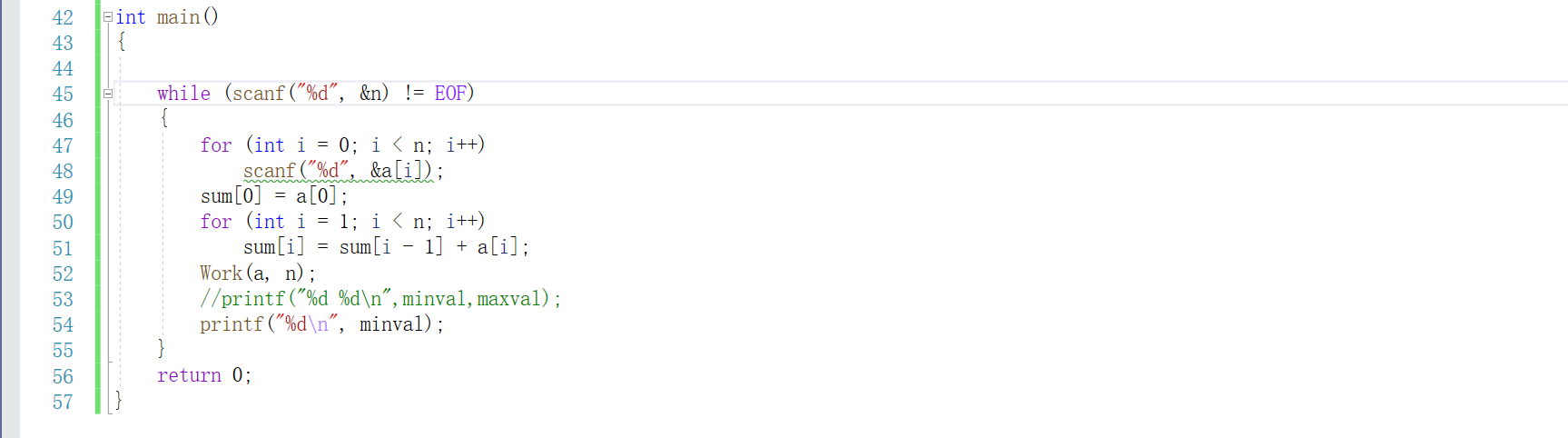#### 伪代码

for i=1 to i=N
for j=i to j=N
计算把第i堆合并到第j堆需要的费用
用sum数组存储
for j=1 to j=n-1
for i=0 to i=n-1
{
对mins[i][j]初始化为无穷大
for k=0 k=j-1
{
计算从i到j共有j-i个状态,取最优值
}
}



#### 图解如下：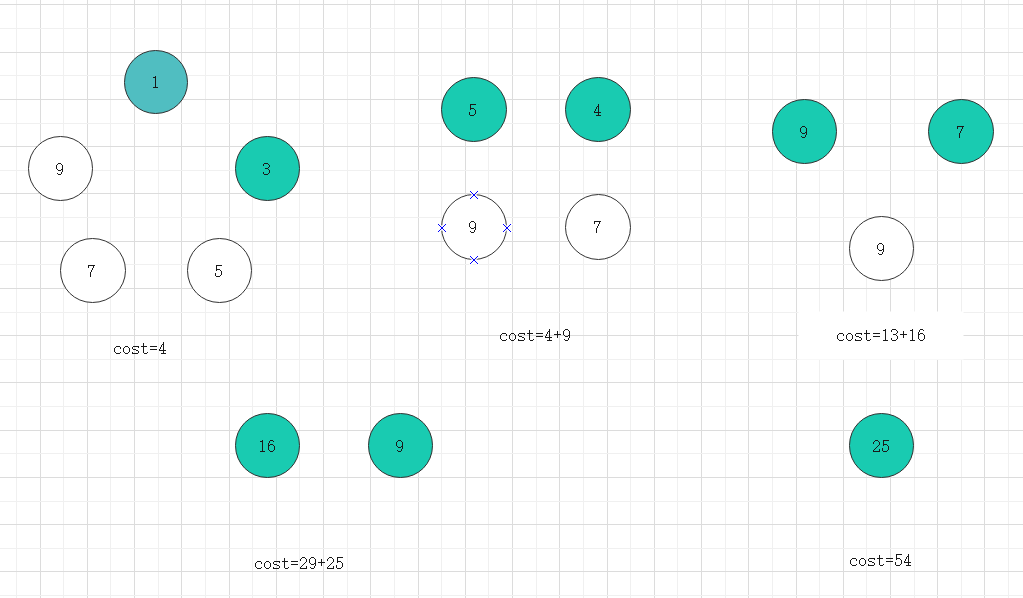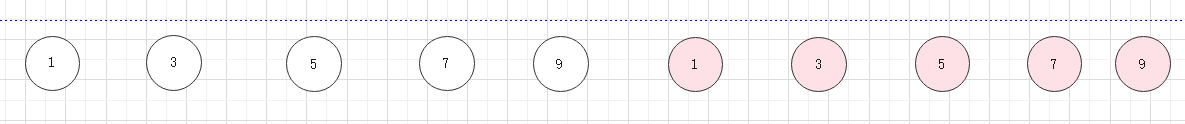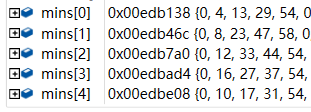• 从第二列开始看
• i=0,j=1,表示第0堆与第一堆进行合并（由于数据存储的时候，是从下标为0开始，但是如果从下标为一 开始，可能更好理解）
• 接下来的一列，都是这个意思
• 从第三列开始看
• i=0，j=2表示将第0堆，第一堆，第二堆进行合并，此时有两种状态，上面也已经说过了
• 总的来说，这道题的思路和上面一道一样，唯一的不同就是处理时，要用%进行求余，可以减少一定的计算时间。

#### 运行结果：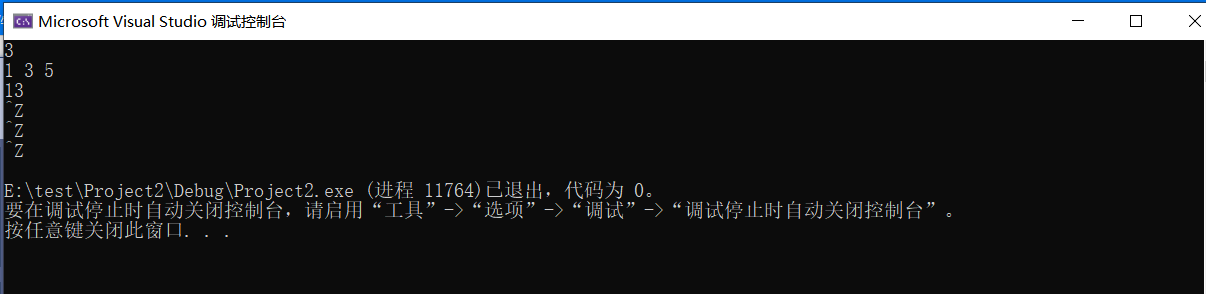#### 题目解题的优势及难点

posted @ 2020-05-06 12:55  是啾咪呀。  阅读(531)  评论(0编辑  收藏  举报